• Astrophysics• Electricity• Electromagnetism• Energy• Fields• Force• Mechanics• Momentum• Nuclear Physics• Quantities & Units• States of Matter• Waves• Key ExperimentsLight is one of the most important things in physics. It has allowed us to create tools that can change the way light travels. Examples of these tools are mirrors and lenses. By combining these tools, we can make telescopes that help us study faraway stars, or microscopes that let us observe tiny living things. To understand how mirrors and lenses work, we use something called ray diagrams. These diagrams help us see how light moves, the route it takes, and how it makes the pictures we see. Let's take a closer look at ray diagrams and how we use them. Ray diagrams are very useful in physics!

## What Does Ray Diagram Mean?

Ray diagrams are an important tool in physics for understanding how light behaves. These diagrams allow us to visualise the path that light takes as it moves from one point to another, such as when it is reflected off a mirror or moves through a lens. They are a simplified representation of light that help us predict where it will end up and how it will form the images we see. Think of it as a hand-drawn simulation of light's movement.

In a ray diagram, the initial point represents the source of light, while the ending point represents the observer's position. Each ray represents a beam of light, and they are drawn following specific rules that depend on the geometry and properties of the object that the ray encounters on its way. While the study of light can be complex, a ray diagram allows us to simplify the process by focusing on the essential details of how light rays interact with different surfaces and materials.

By using ray diagrams, we can gain a deeper understanding of how light works and how it can be manipulated to our advantage. It's a powerful tool that has helped us to create tools like telescopes and microscopes, which have revolutionised the way we study the world around us.

## The Function of Ray Diagrams

It's a fair question to ask why we use ray diagrams when they are an oversimplification of how light actually works. The truth is, by simplifying the study of light, we can focus on the fundamental elements that are necessary to predict its behavior in specific situations.

By abstracting ourselves from unnecessary details such as how much light is absorbed or reflected by a mirror, or the material of a lens, we can predict how light will behave in a given scenario. Although ray diagrams leave out many details, they are still very useful in describing multiple situations, including differently shaped mirrors, diverging and converging lenses, and even complex lens systems like microscopes and telescopes.

So while ray diagrams may not be a complete representation of how light behaves, they are still a valuable tool in simplifying the study of light and predicting its behavior in a variety of situations.

## The Light Ray Diagram

Ray diagrams can sometimes also be called light ray diagrams. Don't worry if you see a question asking you about one or the other, they mean the same thing! Light ray diagrams will always follow some basic rules, no matter if the diagram is for a pinhole camera, a mirror, or a convex lens: Every ray must be drawn as a straight line Every ray must have an arrowhead pointing in the direction that the light is travelling. Since ray diagram can represent multiple situations, more rules are applied depending on the specific case. Let's explore an example of a ray diagram for a plane mirror, so that you can see what a ray diagram looks like. The diagram below is a ray diagram showcasing the reflection of light in a plane mirror.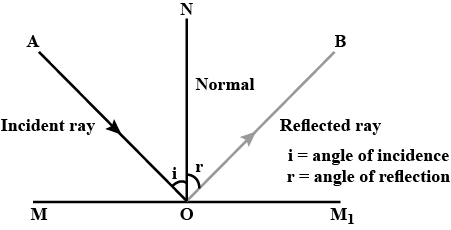This ray diagram of a plane mirror shows how light rays reflect with a reflecting angle equal to the angle of incidence

The first orange line represents the light ray travelling from the light source towards the mirror. Note that as light travels through the same medium it follows a straight line, so make sure you always use a ruler to draw the light rays! This ray diagram is one of the simplest, as it only involves one ray. When we start to look at ray diagrams of lenses, we will need to draw multiple rays, but more on that later. There is one main rule to draw the ray diagram of a mirror: when light reflects, the angle of incidence is always equal to the angle of reflection. In the diagram above, the angle of incidence is marked a sand the angle of reflection is marked as. To identify them we first draw a normal line. The normal line is an imaginary line that extends perpendicular to the surface of the mirror, from the point where the light ray is incident to it. The angle of incidence is angle formed between the incident ray and the normal. The angle of reflection is  angle between the reflected ray and the normal.

We've looked at a regular plane mirror. Let's now consider a particular array of mirrors that can be extremely useful: an L-shaped mirror. Below is a diagram for an L-shaped mirror: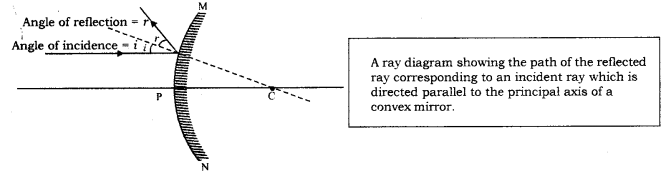The ray diagram for an L-shaped shows how a ray is reflected back, parallel to the incident ray

Notice that there are two locations where reflection occurs. This causes the ray of light from the source to be reflected back in the same direction the ray had when it was incoming. This phenomenon is called retroreflection and it work regardless of the angle of incidence. Moreover, we can scale up this set up by adding a third mirror perpendicular to these two. The result is a mirror with the shape of the corner of a cube. In this three dimensional array any light ray is always reflected directly back to its source. Did you know that in 1969 as part of the Apollo 11 moon mission, astronauts were asked to leave a panel with retroreflectors? This was done so that we could aim a laser from the earth at them and guarantee that light would bounce exactly back in the same direction. Some of the most precise measurements of the distance between the moon and the earth we have to this day were obtained with this method. This is a very impactful experiment and it can be understood with a relatively simple ray diagram! A panel with retroreflectors was deploy to reflect a laser beam back  to its source on earth. NASA

## Convex Lens Ray Diagram

A lens is any shaped piece of material that refracts light in a specific way.Usually, lenses are made from glass, but they can also be made from any transparent material, for example, plastic or even ice! As a quick recap, lenses are a type of object that refracts light, but what does that mean? Refraction is the process where light changes direction when it enters or leaves a new medium, and it takes place because light has a different speed in those mediums.When the light goes through a water-air interface, it changes its direction. This is why an object looks like it is bent when it is partially submerged in a glass of water. The light coming from the submerged part seems to come from a different position than it really is.A pen appears to be bent or broken when partially submerged in water, Kunal B Mehta CC BY-SA 4.0As parallel light rays propagating through the air enter a convex lens they get refracted coming together at a single point.  A convex lens is a lens that is thicker in the middle than at the edges and concentrates light rays at a single point called the principal focus. This is why convex lenses are also called converging lenses.For a regular convex lens, the principal focus will always be along the principal axis.The principal axis is an imaginary horizontal line that goes through the geometric centre of a lens.Notice that the light refracts twice. The first time the light refracts is when it moves from the air into the lens, and then once again as they leave the lens. This is not a ray diagram, when we draw ray diagrams we consider that light rays will only refract at one point, and as such, we can use a much simpler representation for a converging lens. See below for the ray diagram for a convex lens.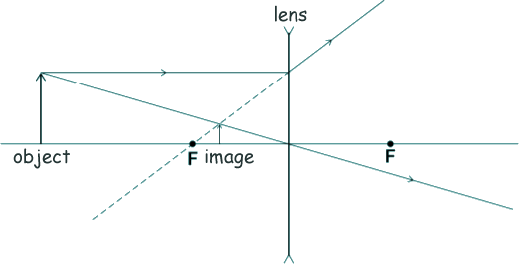In a ray diagram, a convex lens is represented as a vertical line segment with two arrows pointing upwards in the extremes

In this ray diagram, notice that we have labelled both of the foci as  and  to allow us to distinguish between each of them. Convex lenses can work in either direction, so if you had a series of parallel light rays hitting the lens from the right-hand side they would come together at the focus on the left-hand side. Instead of analysing how each light ray will refract, we can get a good understanding of the behaviour of the light rays that go through a convex lens by using the following three special cases: A light ray parallel to the principal axis passes through the focus on the other side of the lens after refracting. A light ray that goes through the optical centre does not have any deflection. A light ray passing through the focus moves parallel to the principal axis after refraction.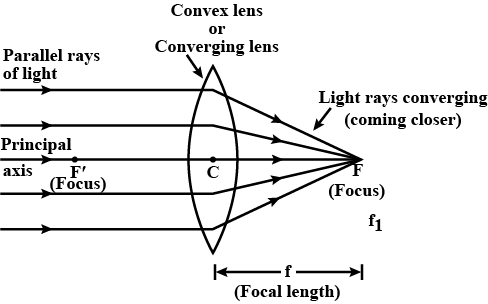A light ray parallel to the principal axis, a ray passing through the centre of the lens, and a ray passing through the focus are special cases that refract in a predictable manner

## Concave Lens Ray Diagram

Besides convex lenses, the other main type of lens that you need to be familiar with is the concave lens.

A concave lens is a diverging lens that causes light rays that are parallel to the principal axis to disperse after they have been refracted by the lens. These rays spread out in such a way that makes them look like they are emerging from a single point called the principal focus of the lens.

You can generally tell if a lens is concave because it is rounded inwards, like a shallow cave in the glass! The following diagram illustrates how light rays passing through a concave lens are dispersed.

As with a convex lens, the light refracts twice, once when entering the lens and once when leaving the lens. However, we can simplify this and create a ray diagram like the one below, which represents the same situation as the image above.

In this ray diagram, notice that the lens is represented by a vertical line segment with two arrowheads pointing inwards.

Similarly to the case of concave lenses, we can simplify the interaction of the light with a concave lens by using the following three special cases:

A light ray after diverging will appear to come from the focus if it is parallel to the principal axis before refracting.  A ray of light will go through the optical centre without any deviation. A ray of light going towards the principal focus will refract and afterwards will move parallel to the principal axis.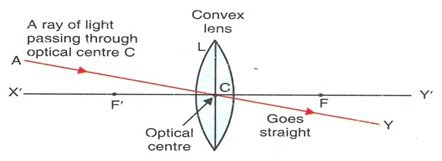In the diagram for a concave lens, light rays passing through the centre do not change direction, but a ray parallel to the principal axis refracts as it came from the focus

We have arrived to the end of this explanation. If you would like to see a more in-depth explanation of ray diagrams to study how images form, and how lenses can magnify images and correct eyesight problems you can take a look at the article on image formation with lenses.

Ray Diagrams - Key takeaways A ray diagram or light ray diagram is a simplified representation of light to study the trajectory that it follows as it moves from one point to another and interacts with different objects that it may encounter on its way. In a ray diagram, a ray represents a beam of light. When drawing a ray diagram, each ray of light must be a straight line. In a ray diagram, a convex lens is illustrated by a line segment with an arrowhead pointing outwards on the ends. A convex lens is a lens that causes rays of light to converge on a point. A concave lens is a lens that causes rays of light to diverge away from each other. In a ray diagram, a convex lens is illustrated by a line segment with an arrowhead pointing outwards on each end. In a ray diagram, a concave lens is illustrated by a line segment with an arrowhead pointing inwards on each end.

## Ray Diagrams

What is a ray diagram?

A ray diagram is a simplified representation of the light that shows the trajectory ray of light from an object to a viewer and shows illustrates how light it interacts with the objects that it may encounter on its way, like mirrors or lenses.

What are the rules of ray diagrams?

The rules depend on the object that the light will interact. For example, there are 3 main rules for drawing a ray diagram of a convex lens: A ray parallel to the principal axis will pass through the focus of a lens. A ray that passes through the focus will become parallel to the principal axis. A ray passing through the optical centre of a lens will come out the other side without any deviation.

How do you draw a ray diagram?

A ray diagram must be drawn with a ruler, as all of the rays of light must be straight lines. This is because light only travels in straight lines. Also, an arrow on the tip of the ray shows the direction of propagation of light. More specific rules depend on whether the light will interact with a mirror or a lense, and their type.

How many ray diagrams are there for a concave mirror?

We can distinguish two main important diagrams for concave mirrors:1. When the object is located farther than the focus of the concave, the image is real, inverted, and reduced in size.2. When the object is located before the focus of a concave mirror, the image is virtual, upright, and enlarged.

What are some examples of ray diagrams?

One of the best examples of applications of ray diagrams is how they can describe image formation by lenses. This allows us to understand how a magnifying glass works.14-day free trial. Cancel anytime.Join 10,000+ learners worldwide.The first 14 days are on us96% of learners report x2 faster learningFree hands-on onboarding & supportCancel Anytime